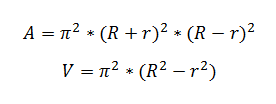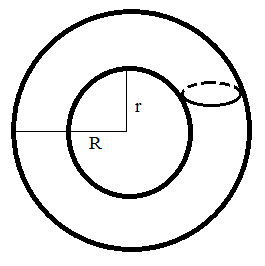# Torus Calculator

This CalcTown calculator calculates the volume and surface area of a torus.

#### Result

m3
m2Click here to view image

Where,

r = inner radius of the torus

R = outer radius of the torus

A = surface area of the torus

V = volume of the torus